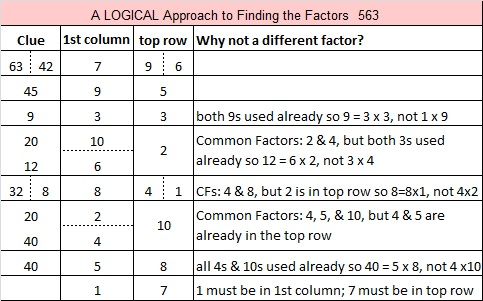# 563 and Level 5

563 is prime, and it is the sum of all the prime numbers from 5 to 67.Print the puzzles or type the solution on this excel file: 10 Factors 2015-07-20

—————————————————————————————————

• 563 is a prime number.
• Prime factorization: 563 is prime.
• The exponent of prime number 563 is 1. Adding 1 to that exponent we get (1 + 1) = 2. Therefore 563 has exactly 2 factors.
• Factors of 563: 1, 563
• Factor pairs: 563 = 1 x 563
• 563 has no square factors that allow its square root to be simplified. √563 ≈ 23.727621How do we know that 563 is a prime number? If 563 were not a prime number, then it would be divisible by at least one prime number less than or equal to √563 ≈ 23.7. Since 563 cannot be divided evenly by 2, 3, 5, 7, 11, 13, 17, 19, or 23, we know that 563 is a prime number.

—————————————————————————————————This site uses Akismet to reduce spam. Learn how your comment data is processed.